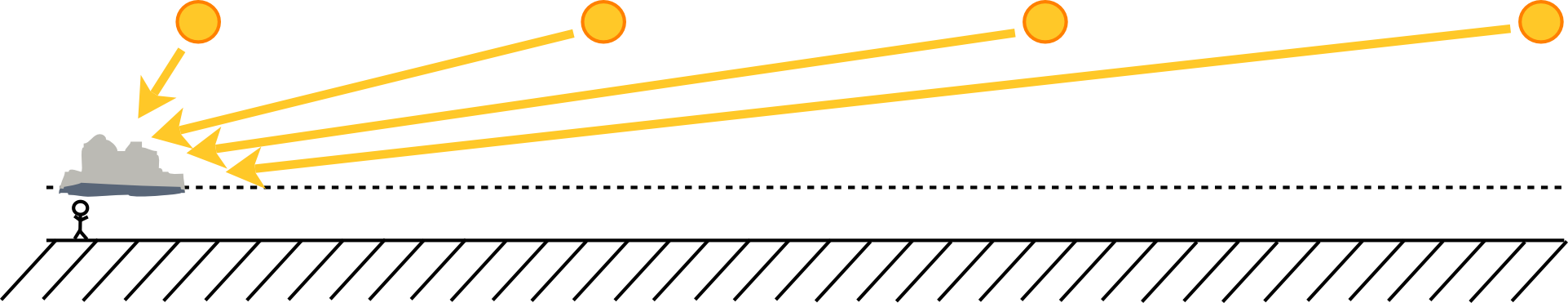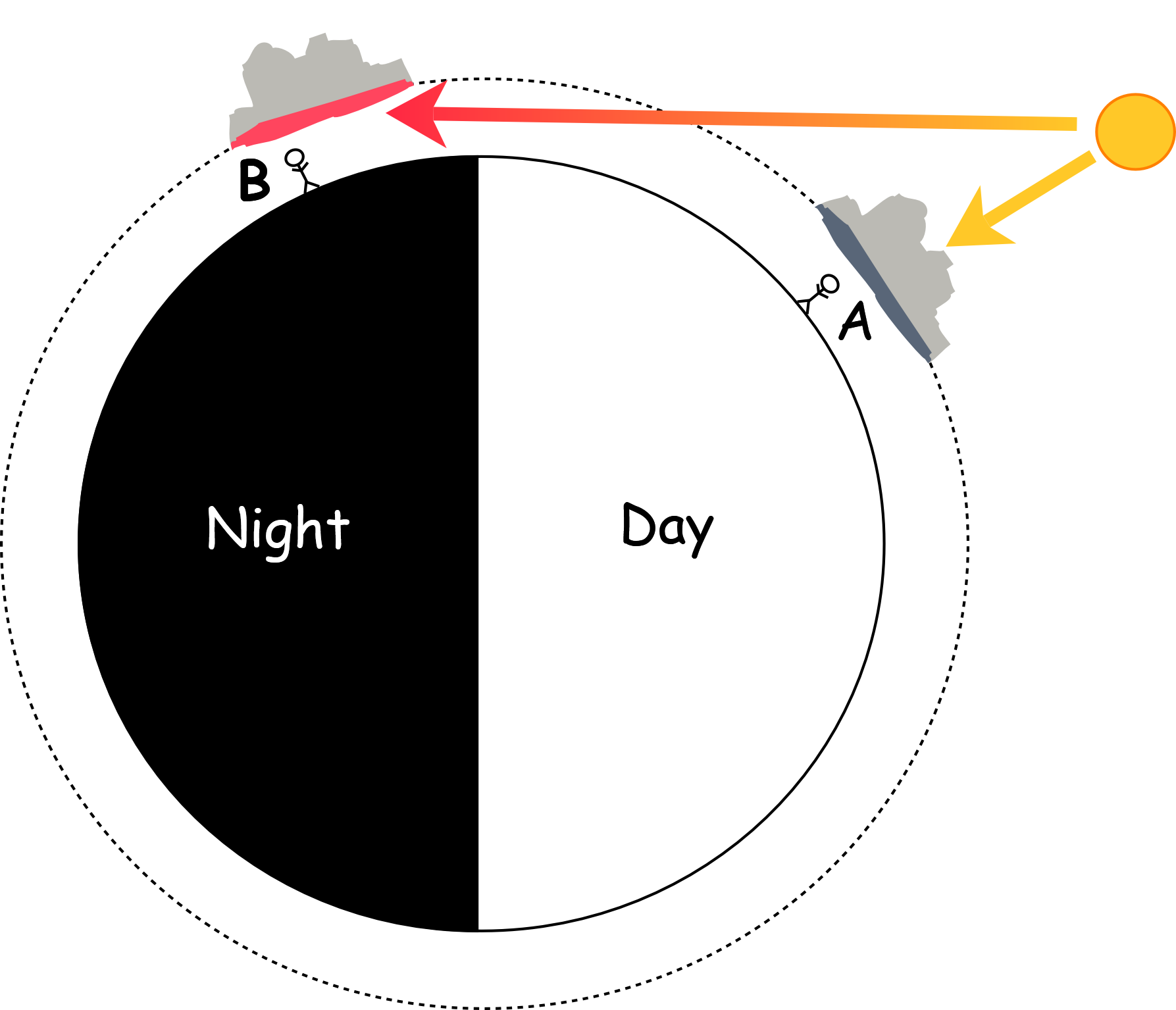# Absolute Simple Proof that the Earth is Round

In recent times there has been an increase in the number of people claiming that the earth is flat.

However, what follows is a simple absolute proof that the earth is spherical without the need of any science or complex arguments.
This is a simple observation that anyone can make at sunrise or sunset with suitable weather conditions.

Round earthers and flat earthers both agree that the sun is higher than the clouds.
In the round earth case, the sun's height 'above' the earth varies from 147 100 000Km and 152 100 000Km.
In the flat earth case, it is suggested the sun is at a height of about 4000Km to 5000Km above the surface of the earth.
Clouds reach as high as the tropopause - typically 12Km above the surface of the earth, so there is no doubt that the sun is always above the clouds.

Now, take a look at this photograph taken by the author. (Full picture [2MB])If the sun is above the clouds, then how is it that the bottom of the cloud is being lit by the sun?
This is impossible with a flat earth as the sun can only be above the cloud regardless of how distant the sun is from the cloud.This is only possible with a round earth as shown in the following diagram:
(Note, the drawing is not to scale.)For observer A, the sun is above the cloud, so the base of the cloud is dark, being shadowed by the rest of the cloud.
For observer B, the sun appears below the base of the cloud because of the curvature of the earth. The sunlight is shining through a long distance of atmosphere giving it the pinky-orange colour (the same reason the moon turns this colour in a total lunar eclipse) and is illuminating the base of the cloud.

A counter to this argument is that the base of the cloud is being lit by a reflection of the sun.
A couple of points to note here, are that even if the sun was being reflected off water, the strength of the reflected light is poor compared to direct sunlight. (The water absorbes light and waves on the surface will scatter the reflection.) The photo above was taken in hilly terrain with no water in the direction of the sun. The terrain would not reflect strong sunlight.

But for sake of this argument, let us assume that the sunlight is being perfectly reflected somehow.
For this obsevation, the sun is on or near the horizon (even below on round earth).
Let us say that the center is 1 degree above the horizon (that is twice the sun's diameter).
Let us also say that the base of the cloud is at 3000m altitude.
Using the diagram below:The sun's light strikes a flat earth at the point o and is reflected bak upto the cloud. The angle the incoming light makes to the surface and the angle the relected light makes to the surface is always the same. Here it is marked a. (This can be tested with a laser beam and a mirror).

To calculate the distance from the point o to the sun, and the base of the cloud, we need to know the height of each and tan(a). [This is the tangent of the angle].
The tan of the angle is defined as the ratio of the vertical distance to the horizontal distance is this diagram. for a right-angled triangle).
So, tan(a) = height / distance.
We need to know the distance so we re-write this as
distance = height / tan(a)
Now we can do this on a calculator.
For the cloud, distance = 3Km / tan( 1 degree ) = 3Km / 0.017455 = 172Km.
For the sun, using the flat earth altitude, distance = 5000Km / tan( 1 degree ) = 5000Km / 0.017455 = 286450Km.
This gives a distance from the cloud to the sun of 286622Km.

And here is a flat earth problem:
The round earth is approximately 40000Km round.
When this is made flat, the flat earth model has the north pole in the center and the south 'pole' forms the rim, 20000Km away, so the diameter of the flat earth is also 40000Km.
According to the various flat earth diagrams, the sun is always above the earth.
So, using the value that flat earthers use, we have shown that the sun can not be illuminating the base of the cloud as it would have to be very far off the edge of the flat earth.

Ironically, the picture below is taken from a flat earth web site:It beautifully proves the earth is round on two accounts:
The sun is on the horizon which is not possible on a flat earth.
Cloud bases nearest the photographer are being lit directly by the sun as it is below them

Flat earthers: Please use the eyes that God gave you!

13. Apr 2018